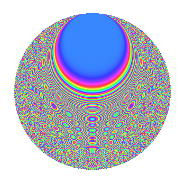Properties

 Label 8049.2.aLevel 8049 Weight 2 Character orbit a Rep. character $$\chi_{8049}(1,\cdot)$$ Character field $$\Q$$ Dimension 447 Newforms 4 Sturm bound 1789 Trace bound 1

Related objects

Defining parameters

 Level: $$N$$ = $$8049 = 3 \cdot 2683$$ Weight: $$k$$ = $$2$$ Character orbit: $$[\chi]$$ = 8049.a (trivial) Character field: $$\Q$$ Newforms: $$4$$ Sturm bound: $$1789$$ Trace bound: $$1$$

Dimensions

The following table gives the dimensions of various subspaces of $$M_{2}(\Gamma_0(8049))$$.

Total New Old
Modular forms 896 447 449
Cusp forms 893 447 446
Eisenstein series 3 0 3

The following table gives the dimensions of the cuspidal new subspaces with specified eigenvalues for the Atkin-Lehner operators and the Fricke involution.

$$3$$$$2683$$FrickeDim.
$$+$$$$+$$$$+$$$$104$$
$$+$$$$-$$$$-$$$$119$$
$$-$$$$+$$$$-$$$$129$$
$$-$$$$-$$$$+$$$$95$$
Plus space$$+$$$$199$$
Minus space$$-$$$$248$$

Trace form

 $$447q$$ $$\mathstrut +\mathstrut q^{2}$$ $$\mathstrut +\mathstrut q^{3}$$ $$\mathstrut +\mathstrut 447q^{4}$$ $$\mathstrut -\mathstrut 2q^{5}$$ $$\mathstrut -\mathstrut 3q^{6}$$ $$\mathstrut +\mathstrut 4q^{7}$$ $$\mathstrut -\mathstrut 3q^{8}$$ $$\mathstrut +\mathstrut 447q^{9}$$ $$\mathstrut +\mathstrut O(q^{10})$$ $$447q$$ $$\mathstrut +\mathstrut q^{2}$$ $$\mathstrut +\mathstrut q^{3}$$ $$\mathstrut +\mathstrut 447q^{4}$$ $$\mathstrut -\mathstrut 2q^{5}$$ $$\mathstrut -\mathstrut 3q^{6}$$ $$\mathstrut +\mathstrut 4q^{7}$$ $$\mathstrut -\mathstrut 3q^{8}$$ $$\mathstrut +\mathstrut 447q^{9}$$ $$\mathstrut -\mathstrut 18q^{10}$$ $$\mathstrut +\mathstrut 4q^{11}$$ $$\mathstrut -\mathstrut q^{12}$$ $$\mathstrut +\mathstrut 2q^{13}$$ $$\mathstrut +\mathstrut 4q^{14}$$ $$\mathstrut -\mathstrut 6q^{15}$$ $$\mathstrut +\mathstrut 451q^{16}$$ $$\mathstrut -\mathstrut 2q^{17}$$ $$\mathstrut +\mathstrut q^{18}$$ $$\mathstrut -\mathstrut 4q^{19}$$ $$\mathstrut +\mathstrut 10q^{20}$$ $$\mathstrut +\mathstrut 4q^{21}$$ $$\mathstrut +\mathstrut 4q^{22}$$ $$\mathstrut +\mathstrut 12q^{23}$$ $$\mathstrut -\mathstrut 15q^{24}$$ $$\mathstrut +\mathstrut 425q^{25}$$ $$\mathstrut +\mathstrut 2q^{26}$$ $$\mathstrut +\mathstrut q^{27}$$ $$\mathstrut -\mathstrut 16q^{28}$$ $$\mathstrut +\mathstrut 2q^{29}$$ $$\mathstrut -\mathstrut 14q^{30}$$ $$\mathstrut +\mathstrut 8q^{31}$$ $$\mathstrut +\mathstrut 5q^{32}$$ $$\mathstrut -\mathstrut 4q^{33}$$ $$\mathstrut -\mathstrut 2q^{34}$$ $$\mathstrut -\mathstrut 8q^{35}$$ $$\mathstrut +\mathstrut 447q^{36}$$ $$\mathstrut +\mathstrut 18q^{37}$$ $$\mathstrut +\mathstrut 12q^{38}$$ $$\mathstrut +\mathstrut 6q^{39}$$ $$\mathstrut -\mathstrut 42q^{40}$$ $$\mathstrut -\mathstrut 10q^{41}$$ $$\mathstrut -\mathstrut 12q^{42}$$ $$\mathstrut -\mathstrut 4q^{43}$$ $$\mathstrut +\mathstrut 60q^{44}$$ $$\mathstrut -\mathstrut 2q^{45}$$ $$\mathstrut +\mathstrut 8q^{46}$$ $$\mathstrut +\mathstrut 16q^{47}$$ $$\mathstrut -\mathstrut q^{48}$$ $$\mathstrut +\mathstrut 459q^{49}$$ $$\mathstrut -\mathstrut q^{50}$$ $$\mathstrut +\mathstrut 2q^{51}$$ $$\mathstrut -\mathstrut 14q^{52}$$ $$\mathstrut +\mathstrut 18q^{53}$$ $$\mathstrut -\mathstrut 3q^{54}$$ $$\mathstrut +\mathstrut 28q^{55}$$ $$\mathstrut +\mathstrut 16q^{56}$$ $$\mathstrut +\mathstrut 8q^{57}$$ $$\mathstrut +\mathstrut 14q^{58}$$ $$\mathstrut +\mathstrut 16q^{59}$$ $$\mathstrut -\mathstrut 42q^{60}$$ $$\mathstrut -\mathstrut 2q^{61}$$ $$\mathstrut +\mathstrut 4q^{62}$$ $$\mathstrut +\mathstrut 4q^{63}$$ $$\mathstrut +\mathstrut 443q^{64}$$ $$\mathstrut -\mathstrut 60q^{65}$$ $$\mathstrut +\mathstrut 12q^{66}$$ $$\mathstrut -\mathstrut 24q^{67}$$ $$\mathstrut +\mathstrut 30q^{68}$$ $$\mathstrut -\mathstrut 36q^{70}$$ $$\mathstrut +\mathstrut 28q^{71}$$ $$\mathstrut -\mathstrut 3q^{72}$$ $$\mathstrut +\mathstrut 6q^{73}$$ $$\mathstrut -\mathstrut 2q^{74}$$ $$\mathstrut -\mathstrut q^{75}$$ $$\mathstrut -\mathstrut 4q^{76}$$ $$\mathstrut +\mathstrut 40q^{77}$$ $$\mathstrut -\mathstrut 14q^{78}$$ $$\mathstrut +\mathstrut 48q^{79}$$ $$\mathstrut +\mathstrut 38q^{80}$$ $$\mathstrut +\mathstrut 447q^{81}$$ $$\mathstrut -\mathstrut 46q^{82}$$ $$\mathstrut +\mathstrut 28q^{83}$$ $$\mathstrut +\mathstrut 4q^{84}$$ $$\mathstrut -\mathstrut 24q^{85}$$ $$\mathstrut +\mathstrut 60q^{86}$$ $$\mathstrut -\mathstrut 18q^{87}$$ $$\mathstrut +\mathstrut 28q^{88}$$ $$\mathstrut -\mathstrut 30q^{89}$$ $$\mathstrut -\mathstrut 18q^{90}$$ $$\mathstrut +\mathstrut 28q^{91}$$ $$\mathstrut +\mathstrut 60q^{92}$$ $$\mathstrut -\mathstrut 8q^{94}$$ $$\mathstrut -\mathstrut 4q^{95}$$ $$\mathstrut -\mathstrut 63q^{96}$$ $$\mathstrut +\mathstrut 2q^{97}$$ $$\mathstrut +\mathstrut 41q^{98}$$ $$\mathstrut +\mathstrut 4q^{99}$$ $$\mathstrut +\mathstrut O(q^{100})$$

Decomposition of $$S_{2}^{\mathrm{new}}(\Gamma_0(8049))$$ into irreducible Hecke orbits

Label Dim. $$A$$ Field CM Traces A-L signs $q$-expansion
$$a_2$$ $$a_3$$ $$a_5$$ $$a_7$$ 3 2683
8049.2.a.a $$95$$ $$64.272$$ None $$-9$$ $$95$$ $$-15$$ $$-36$$ $$-$$ $$-$$
8049.2.a.b $$104$$ $$64.272$$ None $$-9$$ $$-104$$ $$-15$$ $$-10$$ $$+$$ $$+$$
8049.2.a.c $$119$$ $$64.272$$ None $$11$$ $$-119$$ $$17$$ $$10$$ $$+$$ $$-$$
8049.2.a.d $$129$$ $$64.272$$ None $$8$$ $$129$$ $$11$$ $$40$$ $$-$$ $$+$$

Decomposition of $$S_{2}^{\mathrm{old}}(\Gamma_0(8049))$$ into lower level spaces

$$S_{2}^{\mathrm{old}}(\Gamma_0(8049)) \cong$$ $$S_{2}^{\mathrm{new}}(\Gamma_0(2683))$$$$^{\oplus 2}$$